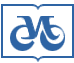Publishing House SB RAS:

Address of the Publishing House SB RAS:
Morskoy pr. 2, 630090 Novosibirsk, Russia

Home – Home – Jornals – Siberian Journal of Numerical Mathematics 2013 number 3

Siberian Journal of Numerical Mathematics

2013 year, number 3

1.Enumeration problems of sets of increasing and decreasing n-valued serial sequences with double-ended constraints on series heights

V.A. Amelkin
Keywords: serial sequences, series length, series height, constraints

Abstract >>
Enumeration problems for n-valued serial sequences are considered. Sets of increasing and decreasing sequences whose structure is specified by constraints on lengths of series and on a difference in heights of the neighboring series in the case when this difference lies between δ1 and δ2 are examined. Formulas for powers of these sets and algorithms for the direct and reverse numerations (assigning smaller numbers to the lexicographically lower-order sequences or smaller numbers to the lexicographically higher-order sequences) are obtained.

2.Finding the most probable non-negative solution of systems of linear algebraic equations by the likelihood method

V.S. Antyufeev
Keywords: system of linear equations, non-negative regularization, probability distribution, stochastic ensemble

Abstract >>
A probabilistic method for regularization is proposed. This method enables one to obtain a non-negative solution to systems of linear algebraic equations. A theorem of existence of the best possible solution is proved. A numerical example of the method application is given.

3.Minimization of nonlinear functions with linear constraints

G.I. Zabinyako, E.A. Kotel'nikov
Keywords: nonlinear programming, reduced gradient, method of conjugate gradients, quasi-Newton method, subgradient method, basis, superbasis

Abstract >>
In this paper, some aspects of numerical realization of algorithms from the software package for solving problems of minimization of nonlinear functions including non-smooth functions with allowance for the linear constraints set by sparse matrices are considered. Examples of the solution of test problems are presented.

4.Variational methods for constructing of monotone approximations for atmospheric chemistry models

V.V. Penenko, E.A. Tsvetova
Keywords: variational principle, stiff systems ODE, integrating multipliers, discrete-analytical approximations, atmospheric chemistry, algorithms for sensitivity studies

Abstract >>
A new method for constructing efficient monotone numerical schemes for solving direct, adjoint, and inverse atmospheric chemistry problems is presented. It is a systhesis of a variational principles combined with splitting and decomposition methods and a constructive realization of the Eulerian integrating factors (EIM) by means of the local adjoint problem technique. To provide the efficiency of calculations, a method to decompose the multi component substances transformation operators in terms of mechanisms of reactions is also proposed. With the analytical EIMs, the decomposed systems of stiff ODEs are reduced to the equivalent systems of integral equations. To solve them, non-iterative multistage algorithms of given order of accuracy are developed. An original variational method for constructing of mutually consistent algorithms for direct and adjoint problems, and sensitivity studies for complex models with constraints is developed.

5.A minimum of the centered discrete random variables dispersion

L.J. Savelyev
Keywords: discrete distribution, random variable, random vector, average value, dispersion

Abstract >>
The problem of isolation of discrete random values and vectors with discrete distributions having a given average value and a minimum dispersion is solved. The vector model is associated with statistical methods of calculation of multiple integrals and solutions to systems of the integral equations.

6.Analytical solution of generalized spectral problem in the method of recalculating boundary conditions for a biharmonic equation

S.B. Sorokin
Keywords: biharmonic equation, boundary conditions, iterative method, Poisson's equation, plate, Dirichlet problem

Abstract >>
An iterative algorithm with an efficient preconditioner for the numerical solution of an elastic problem in approximation of plate theory with mixed boundary conditions is proposed and substantiated. Exact constants of energy equivalence for optimization of iteration method are obtained. Inversion of the preconditioner is equivalent to the double inversion of a discrete analog of the Laplace operator with the Dirichlet boundary conditions.

7.Numerical analytical method of studying some linear functional differential equations

V.B. Cherepennikov
Keywords: functional differential equations, initial value problem, polynomial quasi-solutions, exact solutions

Abstract >>
This paper presents the results of studies of the scalar linear functional-differential equation of a delay type x˙ (t) = a(t)x(t − 1) + b(t)x(t/q) + f(t), q > 1. The main attention is being given to the original problem with the initial point, when the initial condition is specified at the initial point, and the classical solution, whose substitution into the original equation transforms it into the identity, is sought for. The method of polynomial quasi-solution, based on representation of an unknown function x(t) as polynomial of degree N is applied as the method of investigation. Substitution of this function in the original equation results in the residual Delta( t)=O(t^{N}), for which an accurate analytical representation is obtained. In this case, the polynomial quasi-solution is understood as exact solution in the form of polynomial of degree N, disturbed because of the residual of the original initial problem. The theorems of existence of polynomial quasi-solutions for the considered linear functional-differential equation and exact polynomial solutions have been proved. The results of the numerical experiment are presented.

8.Cubic multiwavelets orthogonal to polynomials and a splitting algorithm

B.M. Shumilov
Keywords: hermit splines, multiwavelets, implicit relations of decomposition, parallelization

Abstract >>
In this paper, an implicit method of decomposition of hermit cubic splines using the new type multiwavelets with supercompact supports is investigated. The splitting algorithm of wavelet-transformations on the parallel solution of two three-diagonal systems of the linear equations with strict diagonal domination is reasonable. The results of numerical experiments are presented.